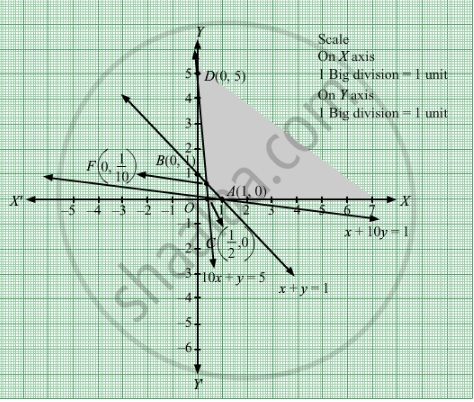# Maximize Z = 2x + 3y Subject to X + Y ≥ 1 10 X + Y ≥ 5 X + 10 Y ≥ 1 X , Y ≥ 0 - Mathematics

Maximize Z = 2x + 3y
Subject to

$x + y \geq 1$
$10x + y \geq 5$
$x + 10y \geq 1$
$x, y \geq 0$

#### Solution

First, we will convert the given inequations into equations, we obtain the following equations:
x y = 1, 10x +y = 5, x + 10y = 1, x = 0 and y = 0
Region represented by x y ≥ 1:
The line x y = 1  meets the coordinate axes at A(1, 0) and B(0,1) respectively. By joining these points we obtain the line x y = 1.
Clearly (0,0) does not satisfies the inequation x y ≥ 1. So,the region in xy plane which does not contain the origin represents the solution set of the inequation x y ≥ 1.
Region represented by 10x +y ≥ 5:
The line 10x +y = 5 meets the coordinate axes at $C\left( \frac{1}{2}, 0 \right)$ and D(0, 5) respectively. By joining these points we obtain the line
10x +y = 5.Clearly (0,0) does not satisfies the inequation 10x +y ≥ 5. So,the region which does not contains the origin represents the solution set of the inequation 10x+y ≥ 5.

Region represented by x + 10y ≥ 1:
The line x + 10y = 1 meets the coordinate axes at $A\left( 1, 0 \right)$ and $F\left( 0, \frac{1}{10} \right)$ respectively. By joining these points we obtain the line
x + 10y = 1.Clearly (0,0) does not satisfies the inequation x + 10y ≥ 1. So,the region which does not contains the origin represents the solution set of the inequation x + 10y ≥ 1.
Region represented by x ≥ 0 and y ≥ 0:
Since, every point in the first quadrant satisfies these inequations. So, the first quadrant is the region represented by the inequations x ≥ 0, and ≥ 0.
The feasible region determined by the system of constraints x y ≥ 1, 10x +y ≥ 5, x + 10y ≥ 1, x ≥ 0, and y ≥ 0, are as follows.The feasible region is unbounded.Therefore, the maximum value is infinity i.e. the solution is unbounded.

Disclaimer:
The obtained answer is for the given question. Answer in the book is 2.It would be 2 if the question is to minimize Z instead of to maximize Z.

Concept: Graphical Method of Solving Linear Programming Problems
Is there an error in this question or solution?

#### APPEARS IN

RD Sharma Class 12 Maths
Chapter 30 Linear programming
Exercise 30.2 | Q 17 | Page 33